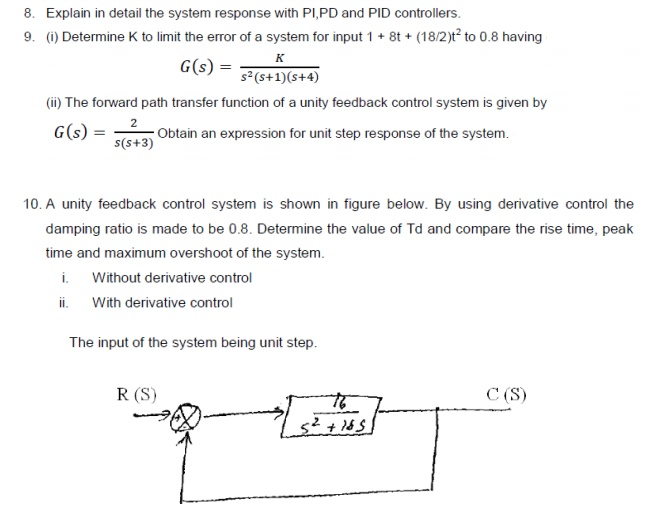Home | | Control System Engineering | Important Short Questions, Answers, Tutorial Problems: Time Response Analysis

# Important Short Questions, Answers, Tutorial Problems: Time Response Analysis

Control Systems - Time Response Analysis - Important Short Questions, Answers, Tutorial Problems: Time Response Analysis

TIME RESPONSE

1.           What is an order of a system?

The order of a system is the order of the differential equation governing the system. The order of the system can be obtained from the transfer function of the given system.

2.           What is step signal?

The step signal is a signal whose value changes from zero to A at t= 0 and remains constant at A for t>0.

3.           What is ramp signal?

The ramp signal is a signal whose value increases linearly with time from an initial value of zero at t=0.the ramp signal resembles a constant velocity.

4.           What is a parabolic signal?

The parabolic signal is a signal whose value varies as a square of time from an initial value of zero at t=0.This parabolic signal represents constant acceleration input to the signal.

5.           What is transient response?

The transient response is the response of the system when the system changes from one state to another.

6.           What is steady state response?

The steady state response is the response of the system when it approaches infinity.

7.           Define Damping ratio.

Damping ratio is defined as the ratio of actual damping to critical Damping.

8.    List the time domain specifications.

The time domain specifications are

i. Delay time

ii. Rise time

iii. Peak time

iv.Peak overshoot

9.           What is damped frequency of oscillation?

In under damped system the response is damped oscillatory. The frequency of damped oscillation is given by ωd = ωn √(1- ζ2)

10. What will be the nature of response of second order system with different types of damping?

For undamped system the response is oscillatory.

For under damped system the response is damped oscillatory.

For critically damped system the response is exponentially rising.

For over damped system the response is exponentially rising but the rise time will be very

large.

11. Define Delay time.

The time taken for response to reach 50% of final value for the very first time is delay

time.

12. Define Rise time.

The time taken for response to raise from 0% to 100% for the very first time is rise time.

13. Define peak time

The time taken for the response to reach the peak value for the first time is peak time.

14. Define peak overshoot.

Peak overshoot is defined as the ratio of maximum peak value measured from the Maximum value to final value

15. Define Settling time.

Settling time is defined as the time taken by the response to reach and stay within specified error

16. What is the need for a controller?

The controller is provided to modify the error signal for better control action.

17. What are the different types of controllers?

The different types of the controller are

Proportional controller

PI controller

PD controller

PID controller

18. What is proportional controller?

It is device that produces a control signal which is proportional to the input error signal.

19. What is PI controller?

It is device that produces a control signal consisting of two terms –one proportional to error signal and the other proportional to the integral of error signal.

20. What is PD controller?

PD controller is a proportional plus derivative controller which produces an output signal consisting of two terms -one proportional to error signal and other proportional to the derivative of the signal.

21. What is the significance of integral controller and derivative controller in a PID controller?

The proportional controller stabilizes the gain but produces a steady state error. The integral control reduces or eliminates the steady state error.

The steady state error is the value of error signal e(t) when t tends to infinity.

23. What is the drawback of static coefficients?

The main drawback of static coefficient is that it does not show the variation of error with time and input should be standard input.

24. What are the three constants associated with a steady state error?

The three steady state errors constant are

Positional error constant Kp

Velocity error constant Kv

Acceleration error constant Ka

25. What are the main advantages of generalized error co-efficients?

i) Steady state is function of time.

ii)  Steady state can be determined from any type of input.

26. What are the effects of adding a zero to a system?

Adding a zero to a system results in pronounced early peak to system response thereby the peak overshoot increases appreciably.

27. Why derivative controller is not used in control system?

The derivative controller produces a control action based on rate of change of error signal and it does not produce corrective measures for any constant error. Hence derivative controller is not used in control system

28. What is the effect of PI controller on the system performance?

The PI controller increases the order of the system by one, which results in reducing the steady state error .But the system becomes less stable than the original system.

29. What is the effect of PD controller on system performance?

The effect of PD controller is to increase the damping ratio of the system and so the peak overshoot is reduced.

30. What is the disadvantage in proportional controller?

The disadvantage in proportional controller is that it produces a constant steady state error.Study Material, Lecturing Notes, Assignment, Reference, Wiki description explanation, brief detail
Control Systems : Time Response Analysis : Important Short Questions, Answers, Tutorial Problems: Time Response Analysis |